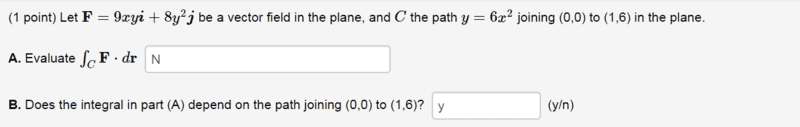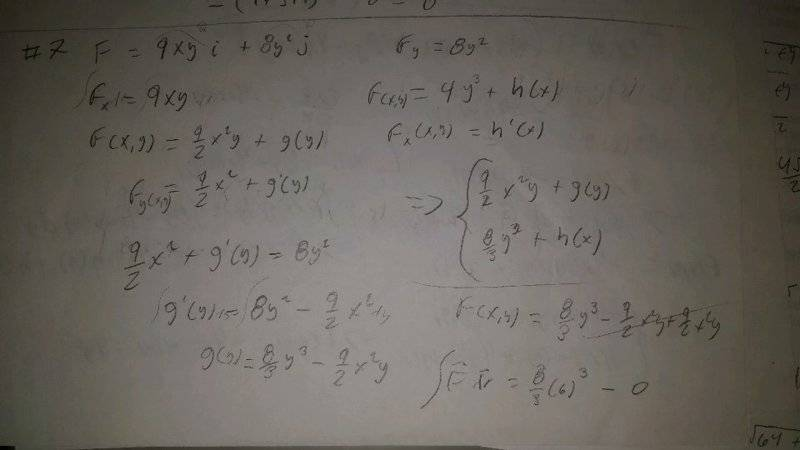# Vector Calculus (non conservative vector fields

the question:My attempt:The partial derivatives did not match so i simply tried to find f(x,y) I got the set of equations on the right but that's about it.

#### Attachments

Homework Helper
Gold Member
## dr=\hat{i} dx+\hat{j} dy ## . With ## y=6x^2 ## it should be a simple matter to evaluate ## \int_C F \cdot dr ## over the path. Do you know how to take a dot product?(This one is in two dimensions instead of three). ## \\ ## For part B, all you need to do is take ## \nabla \times F ## in two dimensions. If it is non-zero, the integral of ## \int_C F \cdot dr ## will be path-dependent.

## dr=\hat{i} dx+\hat{j} dy ## . With ## y=6x^2 ## it should be a simple matter to evaluate ## \int_C F \cdot dr ## over the path. Do you know how to take a dot product?(This one is in two dimensions instead of three).
I do know how to take the dot product but if its a simple matter with y=6x^2 then I must be missing something here. do you take the derivative of y and put it in dy and then solve for x and do the same thing? and then the dot product?

Homework Helper
Gold Member
## \int_C F \cdot dr=\int 9xy \, dx+\int 8y^2 \, dy ##. It doesn't get much easier. You substitute in ## y=6x^2 ## in the first integral. In both integrals, be sure and use the x-limits for the x-integral, and the y-limits for the y.

•jonathanm111
Homework Helper
Gold Member
Additional problem: You can see that the line ## y=6x ## also connects the two endpoints. Try computing ## \int F \cdot dr ## over this straight line path between the same two points. Do you get the same answer? ## \\ ## If not, the integral is clearly path dependent. And if the answer is yes, the integral could still be path dependent for some other paths. The way to prove path dependence or independence is to look at ## \nabla \times F ##.

Last edited:
•jonathanm111
## \int_C F \cdot dr=\int 9xy \, dx+\int 8y^2 \, dy ##. It doesn't get much easier. You substitute in ## y=6x^2 ## in the first integral. In both integrals, be sure and use the x-limits for the x-integral, and the y-limits for the y.
alright i got it to work, its 1179/2.
Additional problem: You can see that the line ## y=6x ## also connects the two endpoints. Try computing ## \int F \cdot dr ## over this straight line path between the same two points. Do you get the same answer? ## \\ ## If not, the integral is clearly path dependent. And if the answer is yes, the integral could still be path dependent for some other paths. The way to prove path dependence or independence is to look at ## \nabla \times F ##.
And you'd get a different answer so its path-dependent. so we could actually use this information for B

•Homework Helper
Gold Member
I had to google the curl in two dimensions=I'm used to working in 3 dimensions. It is simply ## (\frac{\partial{Q}}{\partial{x}}-\frac{\partial{P}}{\partial{y}}) \hat{k} ##, where ## F=P \hat{i}+Q \hat{j} ##. ## \\ ## If you compute it, it should turn out to be non-zero, because you have already demonstrated a path dependence.

last quick question: how did you know to substitute the y into the x-integral

•